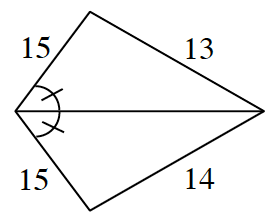### Home > CC4 > Chapter 8 > Lesson 8.2.1 > Problem8-102

8-102.

Carefully examine each diagram below and explain why the geometric figure cannot exist. Support your statements with reasons. Homework Help ✎1.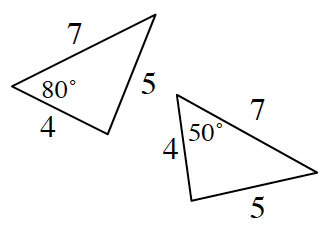Read the Math Notes box from Lesson 7.1.6 to review the triangle congruence conjectures.

The triangles should be congruent by $\text{SSS} ≅$, but $80º ≠ 50º$ and in congruent triangles all angles are equal as well.

1.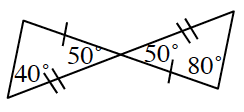The triangles should be congruent by $\text{SAS} ≅$, but $80º ≠ 90º$ and $40º ≠ 50º$ and in congruent triangles all angles are equal as well.

1.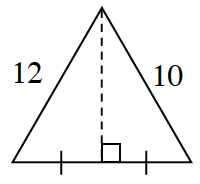The triangles should be congruent by $\text{SAS} ≅$, but $10 ≠ 12$ and in congruent triangles all sides are congruent as well.

1.What do you know about isosceles triangles?

1.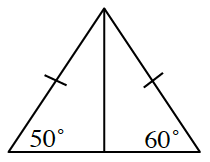1.